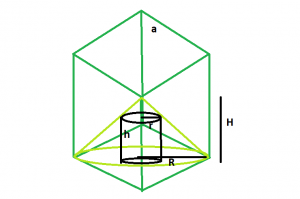# Largest right circular cylinder that can be inscribed within a cone which is in turn inscribed within a cube

Given here is a cube of side length a, which inscribes a cone which in turn inscribes a right circular cylinder. The task is to find the largest possible volume of this cylinder.

Examples:

```Input: a = 5
Output: 232.593

Input: a = 8
Output: 952.699
```## Recommended: Please try your approach on {IDE} first, before moving on to the solution.

Approach:
From the figure, it is very clear, height of cone, H = a and radius of the cone, R = a√2, please refer Largest cone that can be inscribed within a cube.
and, radius of the cylinder, r = 2R/3 and height of the cylinder, h = 2H/3, please refer Largest right circular cylinder that can be inscribed within a cone.
So, radius of cylinder with respect to cube, r = 2a√2/3 and height of cylinder with respect to cube, h = 2a/3.
So, volume of the cylinder, V = 16πa^3/27.

Below is the implementation of the above approach:

## C++

 `// C++ Program to find the biggest right circular  ` `// cylinder that can be inscribed within a right  ` `// circular cone which in turn is inscribed  ` `// within a cube ` `#include ` `using` `namespace` `std; ` ` `  `// Function to find the biggest ` `// right circular cylinder ` `float` `cyl(``float` `a) ` `{ ` ` `  `    ``// side cannot be negative ` `    ``if` `(a < 0) ` `        ``return` `-1; ` ` `  `    ``// radius of right circular cylinder ` `    ``float` `r = (2 * a * ``sqrt``(2)) / 3; ` ` `  `    ``// height of right circular cylinder ` `    ``float` `h = (2 * a) / 3; ` ` `  `    ``// volume of right circular cylinder ` `    ``float` `V = 3.14 * ``pow``(r, 2) * h; ` ` `  `    ``return` `V; ` `} ` ` `  `// Driver code ` `int` `main() ` `{ ` `    ``float` `a = 5; ` `    ``cout << cyl(a) << endl; ` ` `  `    ``return` `0; ` `} `

## Java

 `// Java Program to find the biggest right circular  ` `// cylinder that can be inscribed within a right  ` `// circular cone which in turn is inscribed  ` `// within a cube ` `import` `java.lang.Math; ` ` `  `class` `cfg  ` `{ ` ` `  `// Function to find the biggest ` `// right circular cylinder ` `static` `float` `cyl(``float` `a) ` `{ ` ` `  `    ``// side cannot be negative ` `    ``if` `(a < ``0``) ` `        ``return` `-``1``; ` ` `  `    ``// radius of right circular cylinder ` `    ``float` `r = (``2` `* a *(``float``)(Math.sqrt (``2``)) / ``3``); ` ` `  `    ``// height of right circular cylinder ` `    ``float` `h = (``2` `* a) / ``3``; ` ` `  `    ``// volume of right circular cylinder ` `    ``float` `V =(``3``.14f *(``float``)(Math.pow(r, ``2``) * h)); ` ` `  `    ``return` `V; ` `} ` ` `  `// Driver code ` `public` `static` `void` `main(String[] args) ` `{ ` `    ``float` `a = ``5``; ` `    ``System.out.println(cyl(a)); ` `} ` `} ` ` `  `// This code is contributed by Mukul Singh. `

## Python3

 `# Python3 Program to find the biggest  ` `# right circular cylinder that can be  ` `# inscribed within a right circular  ` `# cone which in turn is inscribed  ` `# within a cube ` `import` `math as mt ` ` `  `# Function to find the biggest ` `# right circular cylinder ` `def` `cyl(a): ` ` `  `    ``# side cannot be negative ` `    ``if` `(a < ``0``): ` `        ``return` `-``1` ` `  `    ``# radius of right circular cylinder ` `    ``r ``=` `(``2` `*` `a ``*` `mt.sqrt(``2``)) ``/` `3` ` `  `    ``# height of right circular cylinder ` `    ``h ``=` `(``2` `*` `a) ``/` `3` ` `  `    ``# volume of right circular cylinder ` `    ``V ``=` `3.14` `*` `pow``(r, ``2``) ``*` `h ` ` `  `    ``return` `V ` ` `  `# Driver code ` `a ``=` `5` `print``(cyl(a)) ` ` `  `# This code is contributed by ` `# Mohit kumar 29 `

## C#

 `// C# Program to find the biggest  ` `// right circular cylinder that can ` `// be inscribed within a right circular ` `// cone which in turn is inscribed  ` `// within a cube  ` `using` `System; ` ` `  `class` `GFG  ` `{  ` ` `  `    ``// Function to find the biggest  ` `    ``// right circular cylinder  ` `    ``static` `float` `cyl(``float` `a)  ` `    ``{  ` ` `  `        ``// side cannot be negative  ` `        ``if` `(a < 0)  ` `            ``return` `-1;  ` ` `  `        ``// radius of right circular cylinder  ` `        ``float` `r = (2 * a * (``float``)(Math.Sqrt (2)) / 3);  ` ` `  `        ``// height of right circular cylinder  ` `        ``float` `h = (2 * a) / 3;  ` ` `  `        ``// volume of right circular cylinder  ` `        ``float` `V =(3.14f * (``float``)(Math.Pow(r, 2) * h));  ` `        ``return` `V;  ` `    ``}  ` ` `  `    ``// Driver code  ` `    ``public` `static` `void` `Main()  ` `    ``{  ` `        ``float` `a = 5;  ` `        ``Console.Write(cyl(a));  ` `    ``}  ` `}  ` ` `  `// This code is contributed by Rajput-Ji  `

## PHP

 ` `

Output:

```232.593
```

Attention reader! Don’t stop learning now. Get hold of all the important DSA concepts with the DSA Self Paced Course at a student-friendly price and become industry ready.

My Personal Notes arrow_drop_upBudding Web DeveloperKeen learnerAverage CoderDancer&Social Activist

If you like GeeksforGeeks and would like to contribute, you can also write an article using contribute.geeksforgeeks.org or mail your article to contribute@geeksforgeeks.org. See your article appearing on the GeeksforGeeks main page and help other Geeks.

Please Improve this article if you find anything incorrect by clicking on the "Improve Article" button below.